#### Distance functions

Many collision detection methods benefit from maintaining a distance function, which keeps track of how far the bodies are from colliding. For example, let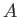and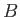denote the set of all points occupied in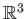by two different models. If they are in collision, then their intersection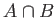is not empty. If they are not in collision, then the Hausdorff distance betweenandis the Euclidean distance between the closest pair of points, taking one fromand one from.8.3 Let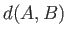denote this distance. Ifandintersect, then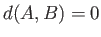because any point inwill yield zero distance. Ifanddo not intersect, then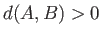, which implies that they are not in collision (in other words, collision free).

Ifis large, thenandare mostly likely to be collision free in the near future, even if one or both are moving. This leads to a family of collision detection methods called incremental distance computation, which assumes that between successive calls to the algorithm, the bodies move only a small amount. Under this assumption the algorithm achieves ``almost constant time'' performance for the case of convex polyhedral bodies [183,218]. Nonconvex bodies can be decomposed into convex components.

A concept related to distance is penetration depth, which indicates how far one model is poking into another . This is useful for setting a threshold on how much interference between the two bodies is allowed. For example, the user might be able to poke his head two centimeters into a wall, but beyond that, an action should be taken.

Steven M LaValle 2020-11-11